NEET  >  Practice Questions: Some Basic Concepts of Chemistry

# Practice Questions: Some Basic Concepts of Chemistry | Chemistry Class 11 - NEET

 1 Crore+ students have signed up on EduRev. Have you?

Basic Concepts of Chemistry

1. The value of Avogadro constant is
(a) 6.022 x 1022 atoms
(b) 6.022 x 1023 atoms
(c) 6.022 x 1023 mol–1
(d) 6.022 x 1022 mol–1
Ans:
c
Solution: Avogadro constant is 6.022 x 1023 mol–1.

2. The number of significant digits in the number 126000 is
(a) 3
(b) 4
(c) 5
(d) 6
Ans:
a
Solution: There are 3 digits. The last three zeros are not significant.

3. The relative atomic mass of sodium is 23. Which of the following statements is not correct about sodium?
(a) Atomic mass of sodium is 23 u.
(b) Atomic mass of sodium is 3.82 x 10-26 kg.
(c) Molar mass of sodium is 23 g mol-1.
(d) The number of atoms in 24 kg of sodium is 6.022 x 1023.
Ans:
d
Solution: The number of atoms in 23 g of Na will be equal to 6.022 x 1023

Question for Practice Questions: Some Basic Concepts of Chemistry
Try yourself:Law of conservation of masses was firstly established by

Question for Practice Questions: Some Basic Concepts of Chemistry
Try yourself:The atomic mass unit is equal to

Question for Practice Questions: Some Basic Concepts of Chemistry
Try yourself:Which of the following expressions is dimensionally correct?

Question for Practice Questions: Some Basic Concepts of Chemistry
Try yourself:Which of the concentration units is temperature dependent?

Question for Practice Questions: Some Basic Concepts of Chemistry
Try yourself:One femtometer stands for

Question for Practice Questions: Some Basic Concepts of Chemistry
Try yourself:The unit of ‘amount of substance’ is

Question for Practice Questions: Some Basic Concepts of Chemistry
Try yourself:Which one of the following is the correct conversion expression of 1 J?

4. The radius of a hydrogen atom is 5.29 X 10-11 m and that of a proton is 1.5 x10-15 m. The ratio of the radius of atom to the radius of proton in the scientific notation will be
(a) 3.526 x 104
(b) 35.266 x 103
(c) 3.5 x 104
(d) 3.526 6667 x103
Ans:
c
Solution: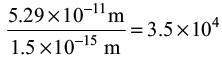5. Mass percent of Na in Na2CO3 is
(a) 21.52%
(b) 31.20%
(c) 38.20%
(d) 43.40%
Ans:
d
Solution: Mass percent of Na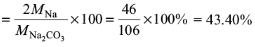6. Select the quantity of NO2 with highest mass:
(a) 100 amu
(b) 1.0 x 10–3 g
(c) 7.0 x 1022 molecules
(d) 8.0 x 10–1 mol
Ans: d
Solution: 100 amu = (100)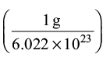= 1.66 x 10–22 g
Mass of 7.0 x 1022 molecules =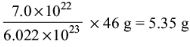Mass of 8.0 x 10–1 mol = 0.8 x 46 g = 36.8 g

Question for Practice Questions: Some Basic Concepts of Chemistry
Try yourself:The volume of concentrated sulphuric acid (98 mass % H2SO4, density 1.84 g cm–3) required to prepare 5 dm3 of 0.5 mol dm–3 solution of sulphuric acid is

Question for Practice Questions: Some Basic Concepts of Chemistry
Try yourself:Iron has a density of 7.86 g cm–3 and an atomic mass of 55.85 amu. The volume occupied by 1 mol of Fe is

Question for Practice Questions: Some Basic Concepts of Chemistry
Try yourself:The combustion of 4.24 mg of an organic compound produces 8.45 mg of CO2 and 3.46 mg of water. The mass percentages of C and H in the compound, respectively, are

Question for Practice Questions: Some Basic Concepts of Chemistry
Try yourself:The simplest formula of the compound containing 32.5% K, 0.839% H, 26.7% S and 39.9% O by mass is

Question for Practice Questions: Some Basic Concepts of Chemistry
Try yourself:If in a reaction HNO3 is reduced to NO, the mass of HNO3 absorbing one mole of electrons would be

Question for Practice Questions: Some Basic Concepts of Chemistry
Try yourself:The SI unit of magnetic flux density is

Question for Practice Questions: Some Basic Concepts of Chemistry
Try yourself:Boron occurs in two varieties, namely, 10B (atomic mass: 10.01 amu) 11B (atomic mass: 11.01 amu). The atomic mass of the naturally occurring element is reported as 10.82 amu. The percent of 10B in this naturally occurring boron is

7. The volume of 0.25 M NaOH to be added to 250 mL of 0.15 M NaOH so that the resultant solution is 0.2 M would be
(a) 250 mL
(b) 350 mL
(c) 450 mL
(d) 550 mL
Ans: a
Solution: Let V be the volume of 0.25 M NaOH solution. Total amount of NaOH after mixing the two solutions is n = V (0.25 mol L–1) + (0.25 L) (0.15 mol L–1)
Total volume of the solution = V + 0.25 L
Molarity of the resultant solution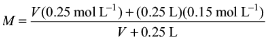Equating this to 0.2 M, we get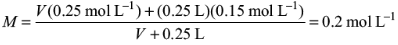Solving for V, we get V = 0.25 L = 250 mL

8. The mass of H2O2 that is completely oxidised by 30.2 g of KMnO4 (molar mass = 158 g mol–1) in acidic medium is
(a) 12 g
(b) 15 g
(c) 17 g
(d) 1 g
Ans:
c
Solution: The reaction is 2KMnO4 + 5H2O2 + 6H+ → 2Mn2+ + 5O2 + 8H2O + 2K+
2 x 151 g of KMnO4 reacts completely with 5 x 34 g of H2O2
30.2 g of KMnO4 reacts completely with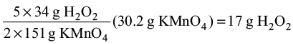Question for Practice Questions: Some Basic Concepts of Chemistry
Try yourself:The sulphur in 1.0 g sample of steel is burned to sulphur dioxide and absorbed in 50.0 mL of 0.1 M sodium hydroxide solution. The excess sodium hydroxide requires 24.0 mL of 0.15 M hydrochloric acid solution for neutralization. The percentage of sulphur in the sample is

Question for Practice Questions: Some Basic Concepts of Chemistry
Try yourself:A 0.50 g sample containing only anhydrous FeCl3 (molar mass: 162.5 g mol-1) and AlCl3 (molar mass: 133.5 g mol-1) yielded 1.435 g of AgCl (molar mass: 143.5 g mol-1). The mass of FeCl3 in the sample is

Question for Practice Questions: Some Basic Concepts of Chemistry
Try yourself:The volume of water needs to be added to 10.0 mL of nitric acid (density: 1.40 g ml-1) containing 70 mass percent of acid to prepare 1.0 M solution would be

The document Practice Questions: Some Basic Concepts of Chemistry | Chemistry Class 11 - NEET is a part of the NEET Course Chemistry Class 11.
All you need of NEET at this link: NEET

## Chemistry Class 11

199 videos|330 docs|229 tests

## Chemistry Class 11

199 videos|330 docs|229 tests

### How to Prepare for NEET

Read our guide to prepare for NEET which is created by Toppers & the best Teachers

Track your progress, build streaks, highlight & save important lessons and more!(Scan QR code)

,

,

,

,

,

,

,

,

,

,

,

,

,

,

,

,

,

,

,

,

,

;